Ex 9.4

Chapter 9 Class 12 Differential Equations
Serial order wise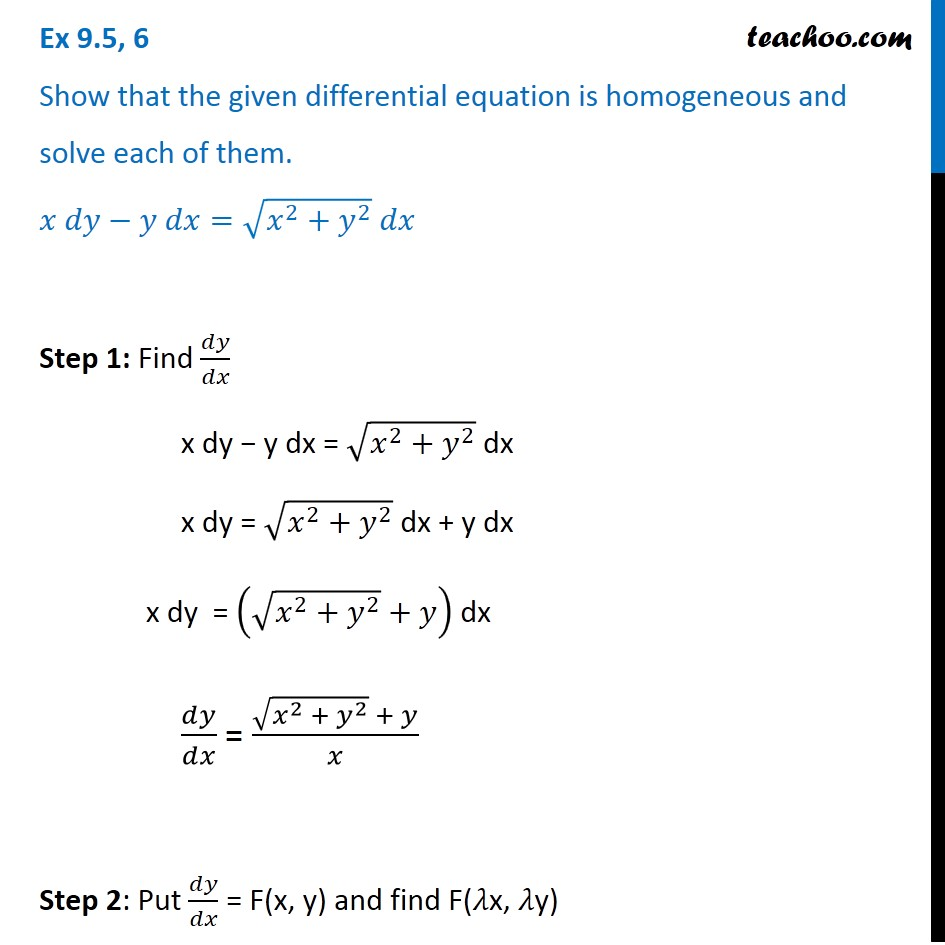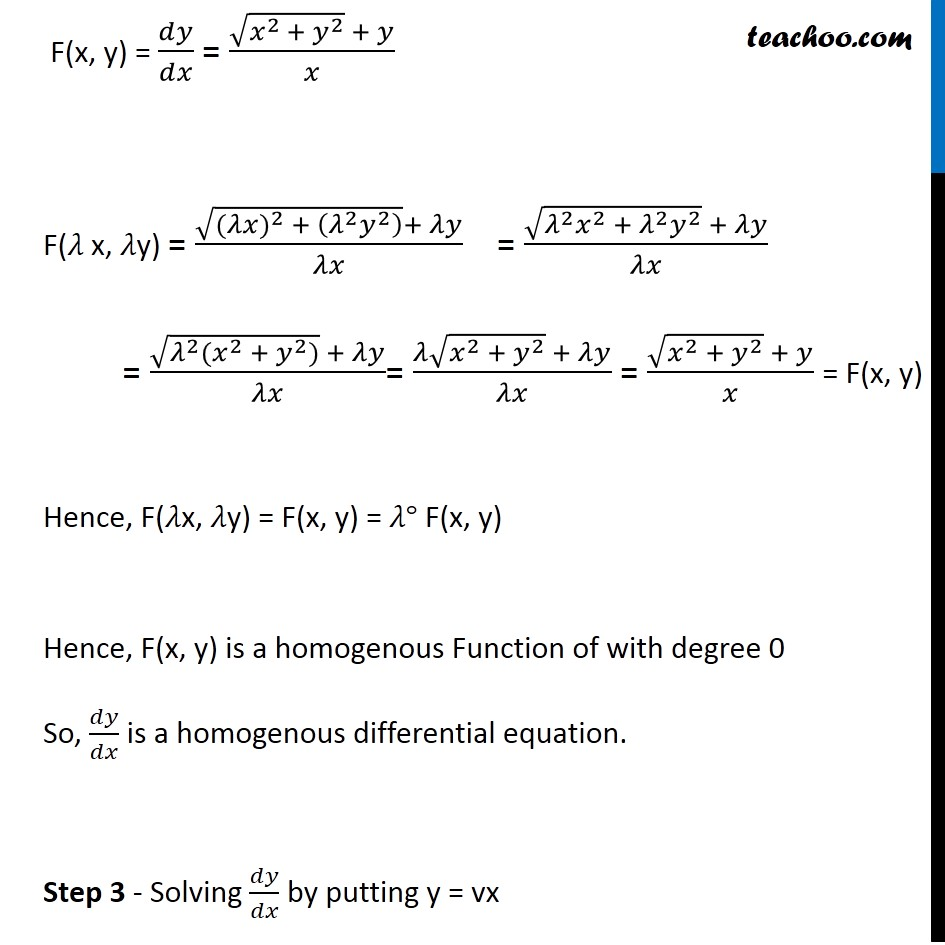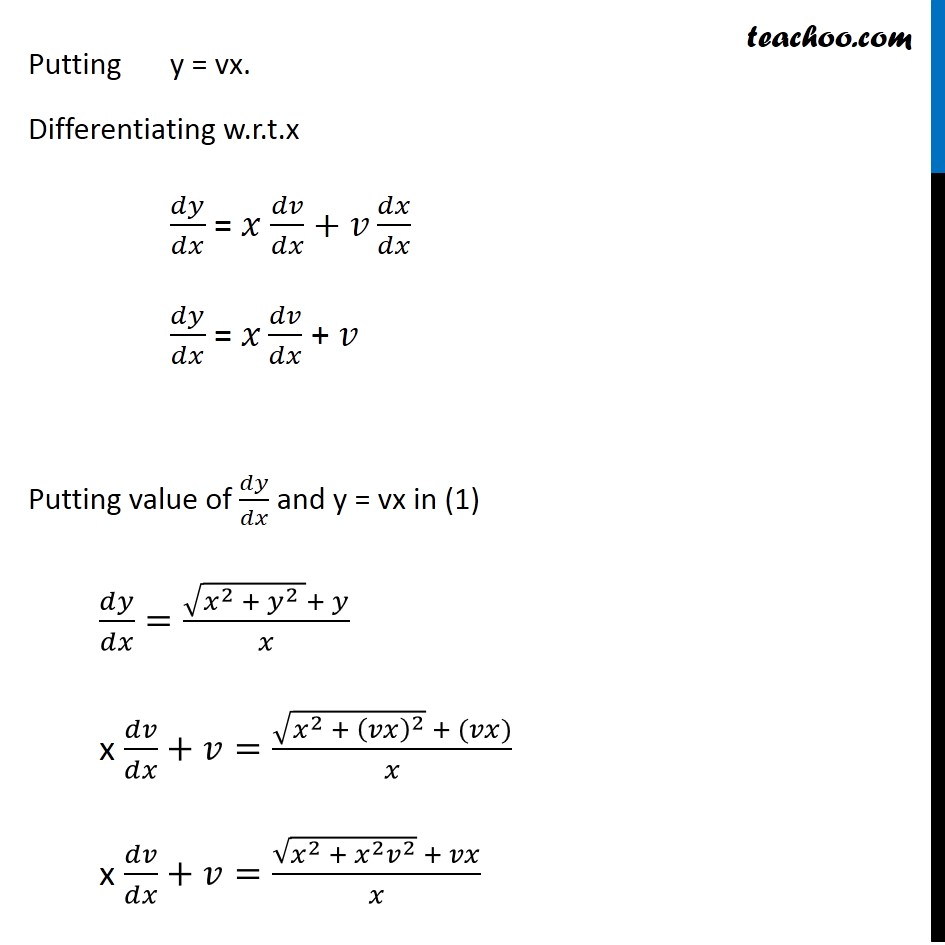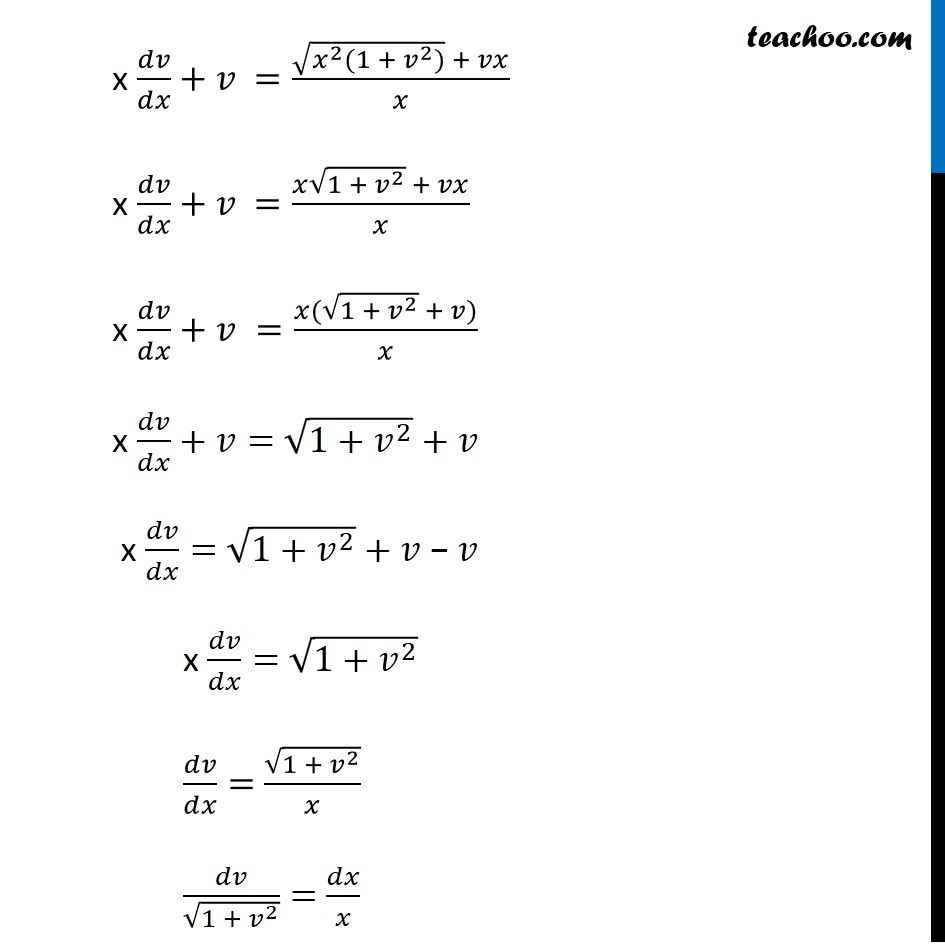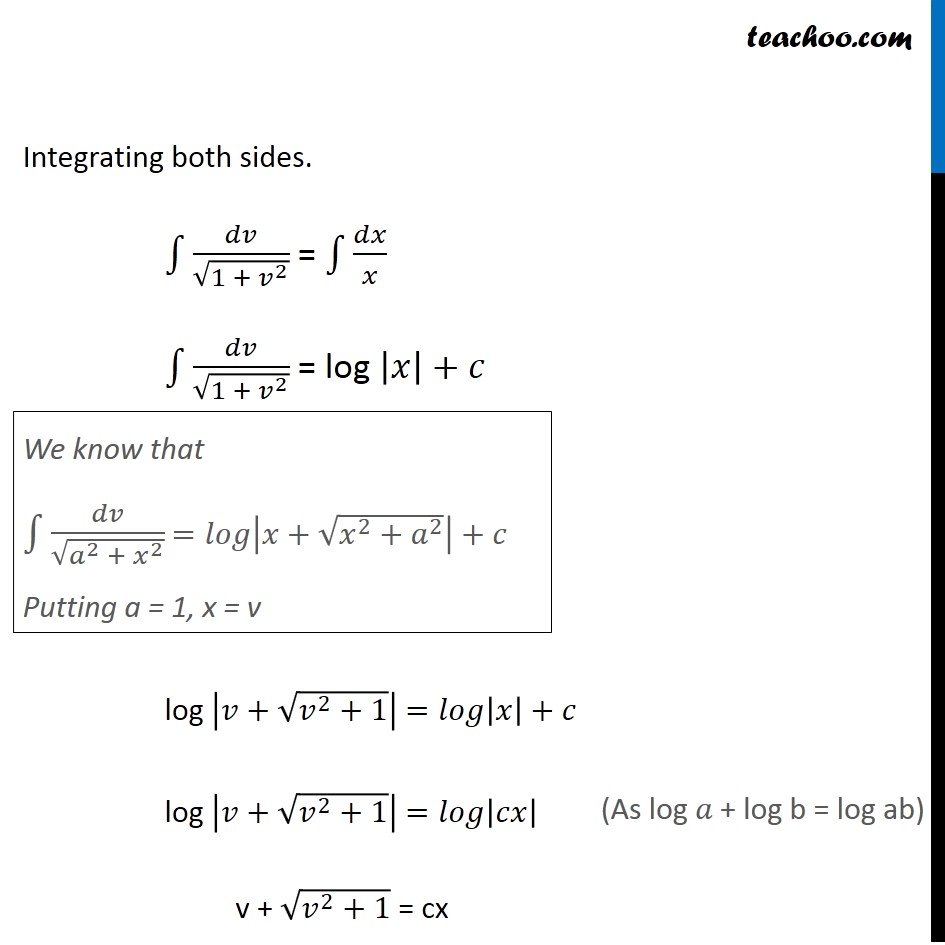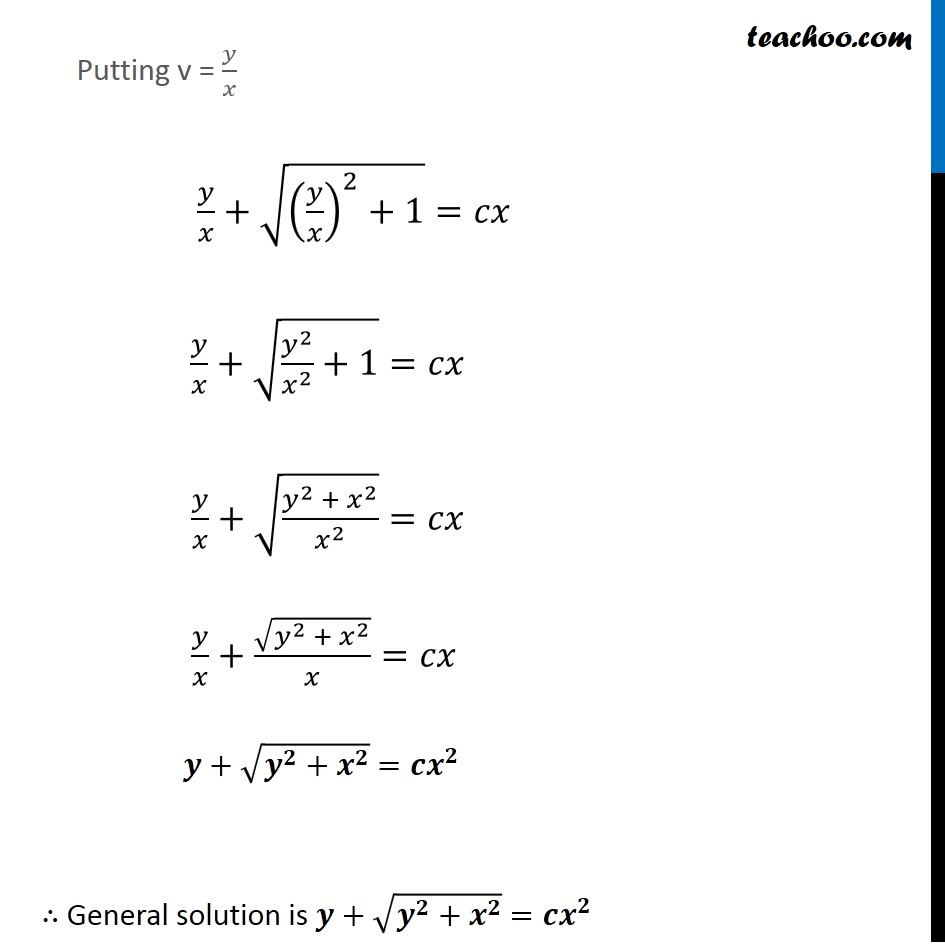Learn in your speed, with individual attention - Teachoo Maths 1-on-1 Class

### Transcript

Ex 9.4, 6 Show that the given differential equation is homogeneous and solve each of them. 𝑥 𝑑𝑦−𝑦 𝑑𝑥=√(𝑥^2+𝑦^2 ) 𝑑𝑥 Step 1: Find 𝑑𝑦/𝑑𝑥 x dy − y dx = √(𝑥^2+𝑦^2 ) dx x dy = √(𝑥^2+𝑦^2 ) dx + y dx x dy = (√(𝑥^2+𝑦^2 )+𝑦) dx 𝑑𝑦/𝑑𝑥 = (√(𝑥^2 + 𝑦^2 ) + 𝑦)/𝑥 Step 2: Put 𝑑𝑦/𝑑𝑥 = F(x, y) and find F(𝜆x, 𝜆y) F(x, y) = 𝑑𝑦/𝑑𝑥 = (√(𝑥^(2 )+ 𝑦^2 ) + 𝑦)/𝑥 F(𝜆 x, 𝜆y) = (√(〖(𝜆𝑥)〗^2 + (𝜆^2 𝑦^2 ) )+ 𝜆𝑦)/𝜆𝑥 = (√(𝜆^2 𝑥^2 + 𝜆^2 𝑦^2 ) + 𝜆𝑦)/𝜆𝑥 = (√(𝜆^2 (𝑥^2 + 𝑦^2)) + 𝜆𝑦)/𝜆𝑥= (𝜆√(𝑥^2 + 𝑦^2 ) + 𝜆𝑦)/𝜆𝑥 = (√(𝑥^2 + 𝑦^2 ) + 𝑦)/𝑥 = F(x, y) Hence, F(𝜆x, 𝜆y) = F(x, y) = 𝜆° F(x, y) Hence, F(x, y) is a homogenous Function of with degree 0 So, 𝑑𝑦/𝑑𝑥 is a homogenous differential equation. Step 3 - Solving 𝑑𝑦/𝑑𝑥 by putting y = vx Putting y = vx. Differentiating w.r.t.x 𝑑𝑦/𝑑𝑥 = 𝑥 𝑑𝑣/𝑑𝑥+𝑣 𝑑𝑥/𝑑𝑥 𝑑𝑦/𝑑𝑥 = 𝑥 𝑑𝑣/𝑑𝑥 + 𝑣 Putting value of 𝑑𝑦/𝑑𝑥 and y = vx in (1) 𝑑𝑦/𝑑𝑥=(√(𝑥^2 + 𝑦^2 )+ 𝑦)/𝑥 x 𝑑𝑣/𝑑𝑥+𝑣=(√(𝑥^2 + (𝑣𝑥)^2 ) + (𝑣𝑥))/𝑥 x 𝑑𝑣/𝑑𝑥+𝑣=(√(𝑥^2 + 𝑥^2 𝑣^2 ) + 𝑣𝑥)/𝑥 x 𝑑𝑣/𝑑𝑥+𝑣 =(√(𝑥^2 (1 + 𝑣^2)) + 𝑣𝑥)/𝑥 x 𝑑𝑣/𝑑𝑥+𝑣 =(𝑥√(1 + 𝑣^2 ) + 𝑣𝑥)/𝑥 x 𝑑𝑣/𝑑𝑥+𝑣 =(𝑥(√(1 + 𝑣^2 ) + 𝑣))/𝑥 x 𝑑𝑣/𝑑𝑥+𝑣= √(1+𝑣^2 )+𝑣 x 𝑑𝑣/𝑑𝑥= √(1+𝑣^2 )+𝑣 − 𝑣 x 𝑑𝑣/𝑑𝑥= √(1+𝑣^2 ) 𝑑𝑣/𝑑𝑥= √(1 + 𝑣^2 )/𝑥 𝑑𝑣/√(1 + 𝑣^2 )= 𝑑𝑥/𝑥 Integrating both sides. ∫1▒𝑑𝑣/√(1 + 𝑣^2 ) = ∫1▒𝑑𝑥/𝑥 ∫1▒𝑑𝑣/√(1 + 𝑣^2 ) = log |𝑥|+𝑐 log |𝑣+√(𝑣^2+1)| =𝑙𝑜𝑔|𝑥|+𝑐 log |𝑣+√(𝑣^2+1)| =𝑙𝑜𝑔|𝑐𝑥| v + √(𝑣^2+1) = cx We know that ∫1▒𝑑𝑣/√(𝑎^2 + 𝑥^2 ) =𝑙𝑜𝑔|𝑥+√(𝑥^2+𝑎^2 )|+𝑐 Putting a = 1, x = v (As log 𝑎 + log b = log ab) Putting v = 𝑦/𝑥 𝑦/𝑥+√((𝑦/𝑥)^2+1)=𝑐𝑥 𝑦/𝑥+√(𝑦^2/𝑥^2 +1)=𝑐𝑥 𝑦/𝑥+√((𝑦^2 + 𝑥^2)/𝑥^2 )=𝑐𝑥 𝑦/𝑥+√(𝑦^2 + 𝑥^2 )/𝑥=𝑐𝑥 𝒚+√(𝒚^𝟐 〖+ 𝒙〗^𝟐 ) =𝒄𝒙^𝟐 ∴ General solution is 𝒚+√(𝒚^𝟐 〖+ 𝒙〗^𝟐 ) =𝒄𝒙^𝟐